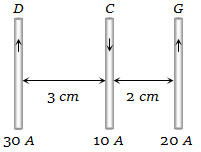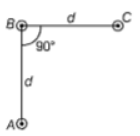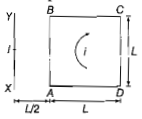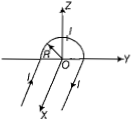Current i is carried in a wire of length L. If the wire is turned into a circular coil, the maximum magnitude of torque in a given magnetic field B will be:

1. $\frac{Li{B}^{2}}{2}$                     2. $\frac{L{i}^{2}B}{2}$

3. $\frac{{L}^{2}iB}{4\mathrm{\pi }}$                     4. $\frac{L{i}^{2}B}{4\mathrm{\pi }}$

Concept Questions :-

Current carrying loop : Force and torque
High Yielding Test Series + Question Bank - NEET 2020

Difficulty Level:

Two thin, long, parallel wires, separated by a distance ‘d’ carry a current of ‘i’ A in the same direction. They will

(a) Attract each other with a force of ${\mu }_{0}{i}^{2}/\left(2{\mathrm{\pi d}}^{2}\right)$

(b) Repel each other with a force of ${\mu }_{0}{i}^{2}/\left(2{\mathrm{\pi d}}^{2}\right)$

(c) Attract each other with a force of ${\mu }_{0}{i}^{2}/\left(2\mathrm{\pi d}\right)$

(d) Repel each other with a force of ${\mu }_{0}{i}^{2}/\left(2\mathrm{\pi d}\right)$

Concept Questions :-

Force between current carrying wires
High Yielding Test Series + Question Bank - NEET 2020

Difficulty Level:

Three long, straight parallel wires carrying current, are arranged as shown in figure. The force experienced by a 25 cm length of wire C is(a) ${10}^{-3}N$

(b) $2.5×{10}^{-3}N$

(c) Zero

(d) $1.5×{10}^{-3}N$

Concept Questions :-

Force between current carrying wires
High Yielding Test Series + Question Bank - NEET 2020

Difficulty Level:

A 250-turn rectangular coil of length 2.1 cm and width 1.25 cm carries a current of 85$\mu$A and subjected to a magnetic field of strength o.85 T. Work done for rotating the coil by ${180}^{\circ }$ against the torque is

(a) 9.1 $\mu J$

(b) 4.55$\mu J$

(c) 2.3$\mu$$J$

(d) 1.5$\mu J$

Concept Questions :-

Magnetic moment
High Yielding Test Series + Question Bank - NEET 2020

Difficulty Level:

An arrangement of three parallel straight wires placed perpendicular to the plane of paper carrying the same current I along the same direction as shown in the figure. Magnitude of force per unit length on the middle wire B is given by:1. $\frac{{\mu }_{o}{i}^{2}}{2\mathrm{\pi d}}$

2. $\frac{2{\mu }_{0}{i}^{2}}{\mathrm{\pi d}}$

3. $\frac{\sqrt{2}{\mu }_{o}{i}^{2}}{\pi d}$

4. $\frac{{\mu }_{o}{i}^{2}}{\sqrt{2}\mathrm{\pi d}}$

Concept Questions :-

Magnetic field due to various cases
High Yielding Test Series + Question Bank - NEET 2020

Difficulty Level:

A long wire carrying a steady current is bent into a circular loop of one turn. The magnetic field at the center of loop is B. It is then bent into a circular coil of n turns. The magnetic field at the centre of this coil of n turns will be

(a) nB                (b) ${n}^{2}B$

(c) 2nB              (d) $2{n}^{2}B$

Concept Questions :-

Magnetic field due to various cases
High Yielding Test Series + Question Bank - NEET 2020

Difficulty Level:

An electron is moving in a circular path under the influence of a transverse magnetic field of $3.57×{10}^{-2}$ T. If the value of e/m is $1.76×{10}^{11}$ C/kg, the frequency of revolution of the electron is

(a) 1 GHz              (b) 100 MHz

(c) 62.8 MHz         (d) 6.28 MHz

Concept Questions :-

Lorentz force
High Yielding Test Series + Question Bank - NEET 2020

Difficulty Level:

A square loop ABCD carrying a current i, is placed near and coplanar with a long straight conductor XY carrying a current I, the net force on the loop will be:1. $\frac{{\mu }_{0}Ii}{2\mathrm{\pi }}$              2. $\frac{2{\mu }_{0}IiL}{3\mathrm{\pi }}$

3. $\frac{{\mu }_{0}IiL}{2\mathrm{\pi }}$            4. $\frac{2{\mu }_{0}Ii}{3\mathrm{\pi }}$

Concept Questions :-

Current carrying loop : Force and torque
High Yielding Test Series + Question Bank - NEET 2020

Difficulty Level:

A long straight wire of radius a carries a steady current I. The current is uniformly distributed over its cross-section. The ratio of the magnetic fields B and B' at radial distances a/2 and 2a respectively, from the axis of the wire, is:

1. 1/2

2. 1

3. 4

4. 1/4

Concept Questions :-

Magnetic field due to various cases
High Yielding Test Series + Question Bank - NEET 2020

Difficulty Level:

A wire carrying current l has the shape as shown in the adjoining figure. Linear parts of the wire are very long and parallel to X-axis while the semicircular portion of radius R is lying in the Y-Z plane. Magnetic field at point O is :1. $\mathrm{B}=\frac{{\mathrm{\mu }}_{0}}{4\mathrm{\pi }}×\frac{\mathrm{i}}{\mathrm{R}}\left(\mathrm{\pi }\stackrel{^}{\mathrm{i}}+2\stackrel{^}{\mathrm{k}}\right)$

2. $\mathrm{B}=-\frac{{\mathrm{\mu }}_{0}}{4\mathrm{\pi }}×\frac{\mathrm{i}}{\mathrm{R}}\left(\mathrm{\pi }\stackrel{^}{\mathrm{i}}-2\stackrel{^}{\mathrm{k}}\right)$

3. $\mathrm{B}=-\frac{{\mathrm{\mu }}_{0}}{4\mathrm{\pi }}×\frac{\mathrm{i}}{\mathrm{R}}\left(\mathrm{\pi }\stackrel{^}{\mathrm{i}}+2\stackrel{^}{\mathrm{k}}\right)$

4. $\mathrm{B}=\frac{{\mathrm{\mu }}_{0}}{4\mathrm{\pi }}×\frac{\mathrm{i}}{\mathrm{R}}\left(\mathrm{\pi }\stackrel{^}{\mathrm{i}}-2\stackrel{^}{\mathrm{k}}\right)$

Concept Questions :-

Magnetic field due to various cases